Function Repository Resource:

Display a Wolfram model description in an analogue of traditional mathematical notation

Contributed by: Stephen Wolfram and Jan Mangaldan
 ResourceFunction["WolframModelTraditionalForm"][rules] converts a Wolfram model represented by rules into a more traditional notation. ResourceFunction["WolframModelTraditionalForm"][rule,"alphabet"] uses letters in “alphabet” to represent the Wolfram model.

## Details and Options

Possible values for "alphabet" include "English" and "Greek".
The number of possible symbols is limited by the number of available symbols in "alphabet".

## Examples

### Basic Examples (2)

A Wolfram model represented in canonical form:

 In:=Out=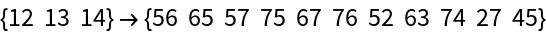The same Wolfram model, but represented with letters:

 In:=Out=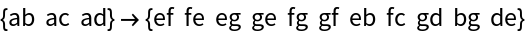### Scope (2)

Use Greek letters to represent the Wolfram model:

 In:=Out=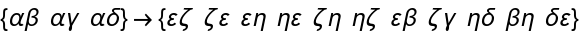Use Hebrew letters:

 In:=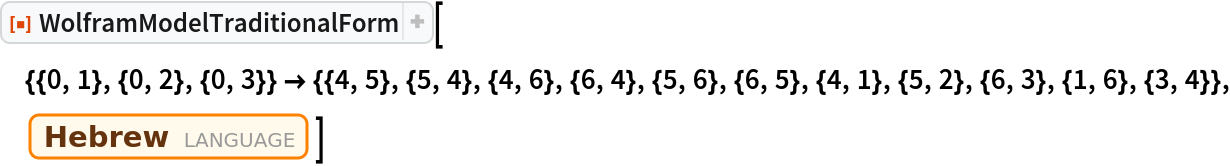Out=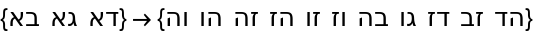## Version History

• 1.0.0 – 16 March 2020